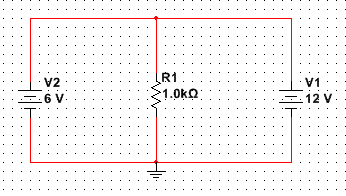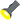# Two different voltage sources in parallel....

cocopops12What would happen if this circuit was implemented practically with batteries?

This circuit makes no logic to me because how can the same node be at two different electric potentials?.

But i tried thinking about it and here's my 'naive' explanation: The 12V battery will start supplying a current of 12/1000 = 12mA, the 6V battery will supply 0 current, until the 12V battery starts to drain and lose its voltage, when the 12V battery reaches 6V it will still be supplying a current of 6mA, but the original 6V battery will start supplying a current of also 6mA, so we will have the same current that was supplied by the 12V (12mA) battery alone the moment the circuit was connected.

If we used DC sources that maintained constant voltages (not batteries) then the 6V source would have no contribution since it won't supply any current.

that's my naive explanation...so tell me what is your explanation?

Studiot
You need to add a resistance in series with each voltage source to correctly model each one.

Then you can correctly solve the circuit.

This is the way parallel voltage sources are calculated in real life - both the voltage and the effective series resistance (ESR) need be known (or measured).

Can you see how to measure the ESR?

cocopops12
You need to add a resistance in series with each voltage source to correctly model each one.

Then you can correctly solve the circuit.

This is the way parallel voltage sources are calculated in real life - both the voltage and the effective series resistance (ESR) need be known (or measured).

Can you see how to measure the ESR?

Thank you sir! does the ESR have to be of a specific value? isn't the natural resistance of the wires is enough?

Studiot
Your circuit cannot be realized with 'ideal' components.

Luckily all real world components are not ideal.

In particular voltage sources always have act as though there is a resistance in series with them.

The lower this resistance, the nearer to ideal the voltage source is and the more powerful it is.

This ESR is specific to an individual voltage source.

So for the largest 1.5 volt battery(D cell) for instance it may be 0.2 ohms.

For the next size (C cell) it may be 0.75 ohms

An AA cell has a couple of ohms and an AAA cell maybe 10 ohms.

cocopops12
Your circuit cannot be realized with 'ideal' components.

Luckily all real world components are not ideal.

In particular voltage sources always have act as though there is a resistance in series with them.

The lower this resistance, the nearer to ideal the voltage source is and the more powerful it is.

This ESR is specific to an individual voltage source.

So for the largest 1.5 volt battery(D cell) for instance it may be 0.2 ohms.

For the next size (C cell) it may be 0.75 ohms

An AA cell has a couple of ohms and an AAA cell maybe 10 ohms.

Thanks , now i understand what was the problem. :)

Staff EmeritusWhat would happen if this circuit was implemented practically with batteries?
Expect one or both of the batteries to seriously overheat, buckle, and possibly explode.
This circuit makes no logic to me because how can the same node be at two different electric potentials?
The higher voltage battery would try to charge the other battery, but the charging current has nothing to limit it to a reasonable level. So both would be dangerously loaded well beyond their ratings, quickly overheat and boil.

•1 person
Gold Member
Expect one or both of the batteries to seriously overheat, buckle, and possibly explode.

The higher voltage battery would try to charge the other battery, but the charging current has nothing to limit it to a reasonable level. So both would be dangerously loaded well beyond their ratings, quickly overheat and boil.

Indeed!

Therefore you would want to isolate the 2 batteries with diodes

to the OP...
But the bigger question is ... WHY would you want to do this, practically it doesn't achieve anything ??

Dave

richard schlesser
will this work say you have 4each 6 volt batteries and 2 each12 volt if you wire your 4 each 6 volts in a series- parallel so the 4each 6 volts are are now in princeable 2 12 volts wired parallel could we now add 2 12 volt batteries into the parallel giveing you a 4 12 volt battery bank

Gold Member
Dearly Missed
that's my naive explanation...so tell me what is your explanation?

You're doing just fine.

In the beginning...

It is important to train ourselves to think with ideal components. Really it's simpler that way. When you get a result of infinity or division by zero you know you need to inject a dose of reality into your thinking.

So
What did they tell you about an ideal voltage source ? They should have told you it has zero internal resistance and is capable of infinite current. They should have told you it will maintain its terminal voltage regardless of current through it.

Applying those behaviors to your circuit elements
i see a voltage source of 12 volts in parallel with one of six volts. Or are they in series ?
writing KVL around the outer loop i get 12 - 6 - ( I X 0) = 0 :<<<(I X 0 is current X resistance around loop)>>>
solve for I
I = (12-6) / 0 which would like to be infinite
so the 12 volt source would like to drive infinite current through the 6 volt one with both of them holding their own
which you know is impossible.

So you have to include resistance of the wires and internal resistance of the batteries in order to solve this one .

Learn to make that mental leap into the ideal and then back off toward reality. It's a fundamental tool you'll use the rest of your engineering life.

old jim

•CalcNerd